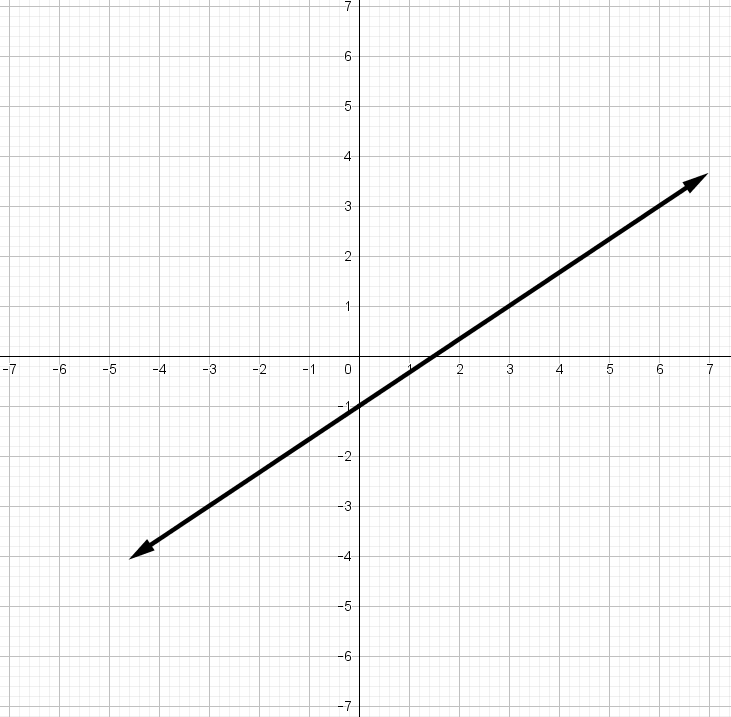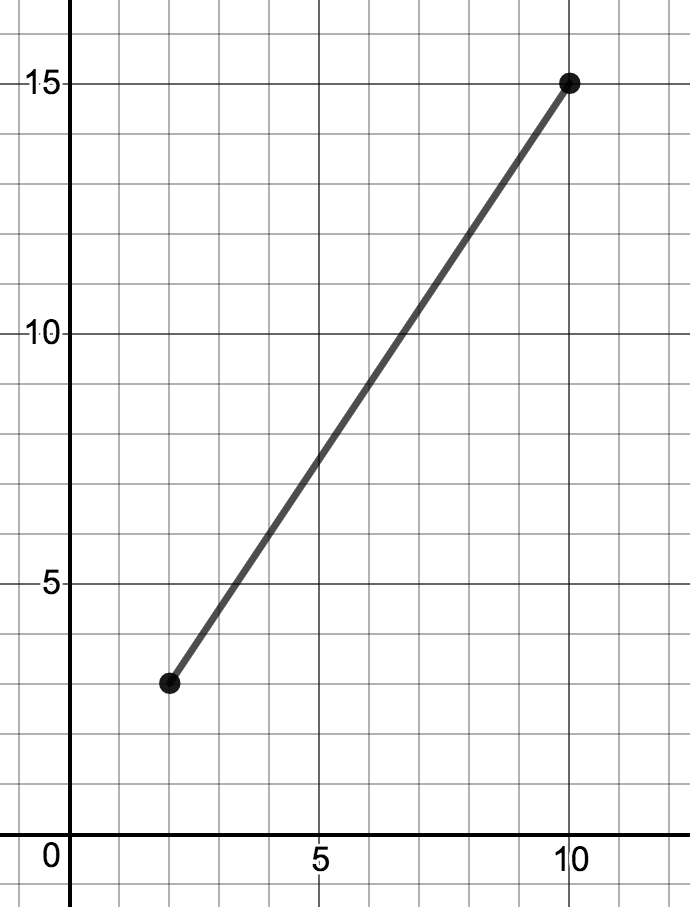# Right Triangles and Trigonometry

## Objective

Describe the relationship between slope and the tangent ratio of the angle of elevation/depression. Use the tangent ratio of the angle of elevation or depression to solve real-world problems.

## Common Core Standards

### Core Standards

?

• G.SRT.C.8 — Use trigonometric ratios and the Pythagorean Theorem to solve right triangles in applied problems. Modeling is best interpreted not as a collection of isolated topics but in relation to other standards. Making mathematical models is a Standard for Mathematical Practice, and specific modeling standards appear throughout the high school standards indicated by a star symbol (★). The star symbol sometimes appears on the heading for a group of standards; in that case, it should be understood to apply to all standards in that group.

?

• 8.EE.B.5

• 8.EE.B.6

## Criteria for Success

?

1. Describe how the slope of a line compares to the tangent of the angle of depression.
2. Use the context of the problem to determine whether the angle is an elevation or a depression.
3. Explain that the ratios of any right angle formed from a single line where the line is the hypotenuse will be equal (slope triangles from 8th grade).
4. Describe that the angle of elevation is congruent to the angle of depression, but they are not the same angle.

## Tips for Teachers

?

• This lesson is an extension of 8.EE.6 by relating slope to the tangent ratio. As a result, students may need to review the concept of slope before they can fully access this lesson. It is recommended to spend time outside of class building this skill.
• This is the first lesson out of two that begins to have students model real-world examples of trigonometric ratios.

## Anchor Problems

?

### Problem 1

Brandon and Madison use different triangles to determine the slope of the line shown below.Brandon started at (0,-1) and drew a right triangle going up 2 units and right 3 units.
Madison started at (-3,-3) and drew a right triangle going up 6 units and right 9 units.

1. Draw and label both triangles on the graph.
2. How can Brandon and Madison use their triangles to find the slope?
3. What trigonometric ratio do Brandon and Madison use to find the slope?

### Problem 2

Below is a line segment on a coordinate grid.1. Draw the right triangle that you can use to find the length of this line segment.
2. Find the length of the line segment.
3. Find the measure of all of the angles of the triangle formed.

### Problem 3

Standing on the gallery of a lighthouse (the deck at the top of a lighthouse), a person spots a ship at an angle of depression of 20°. The lighthouse is 28 meters tall and sits on a cliff 45 meters tall as measured from sea level.  What is the horizontal distance between the lighthouse and the ship?

#### References

EngageNY Mathematics Geometry > Module 2 > Topic E > Lesson 29Exercise 1

Geometry > Module 2 > Topic E > Lesson 29 of the New York State Common Core Mathematics Curriculum from EngageNY and Great Minds. © 2015 Great Minds. Licensed by EngageNY of the New York State Education Department under the CC BY-NC-SA 3.0 US license. Accessed Dec. 2, 2016, 5:15 p.m..

Modified by The Match Foundation, Inc.

## Problem Set

?

The following resources include problems and activities aligned to the objective of the lesson that can be used to create your own problem set.

• Include word problems where students are required to draw a diagram with the angle of elevation or depression.